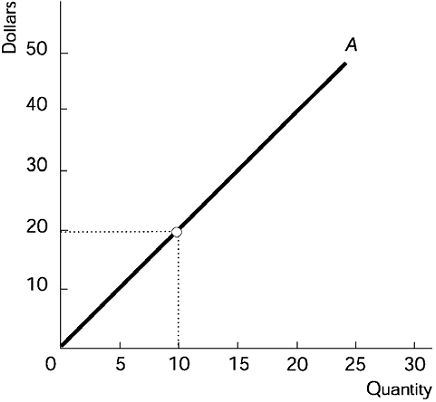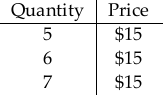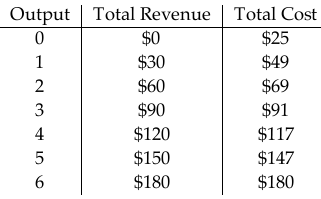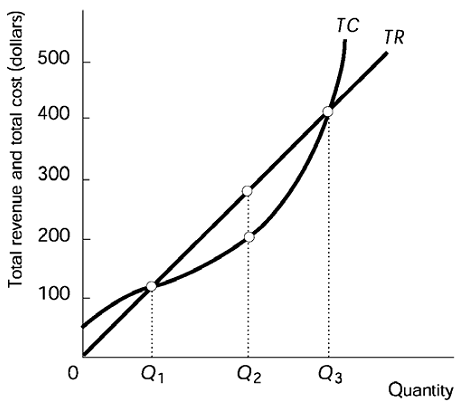Bài sắp đăng
Home | Trắc nghiệm | Kinh tế học | Trắc nghiệm Kinh tế Vi mô – C11 (EN)Ngân hàng câu hỏi trắc nghiệm Kinh tế Vi mô

# Trắc nghiệm Kinh tế Vi mô – C11 (EN)

1. Perfect competition is an industry with
A. a few firms producing identical goods.
B. many firms producing goods that differ somewhat.
C. a few firms producing goods that differ somewhat in quality.
D. many firms producing identical goods.

Correct: D

2. In a perfectly competitive industry, there are
A. many buyers and many sellers.
B. many sellers, but there might be only one or two buyers.
C. many buyers, but there might be only one or two sellers.
D. one firm that sets the price for the others to follow.

Correct: A

3. In perfect competition, the product of a single firm
A. is sold to different customers at different prices.
B. has many perfect complements produced by other firms.
C. has many perfect substitutes produced by other firms.
D. is sold under many differing brand names.

Correct: C

4. In perfect competition, restrictions on entry into an industry
A. do not exist.
B. apply to labor but not to capital.
C. apply to both capital and labor.
D. apply to capital but not to labor.

Correct: A

5. In perfect competition,
A. there are significant restrictions on entry.
B. each firm can influence the price of the good.
C. there are few buyers.
D. all firms in the market sell their product at the same price.

Correct: D

6. The price elasticity of demand for any particular perfectly competitive firm’s output is
A. less than 1.
B. equal to zero.
C. infinite.
D. 1.

Correct: C

7. The demand for wheat from farm A is perfectly elastic because wheat from farm A is a(n)
A. perfect complement to wheat from farm B.
B. perfect substitute for wheat from farm B.
C. normal good.
D. inferior good.

Correct: B

8. In perfect competition, the elasticity of demand for the product of a single firm is
A. 0.
B. infinite.
C. 1.
D. between 0 and 1.

Correct: B

9. In perfect competition, the elasticity of demand for the product of a single firm is
A. infinite, because many other firms produce identical products.
B. zero, because many other firms produce identical products.
C. zero, because the firm produces a unique product.
D. infinite, because the firm produces a unique product.

Correct: A

10. In perfect competition, an individual firm
A. has a price elasticity of supply equal to one.
B. faces unitary elasticity of demand.
C. has a price elasticity of supply equal to infinity.
D. faces infinitely elastic demand.

Correct: D

11. If Steve’s Apple Orchard, Inc. is a perfectly competitive firm, the demand for Steve’s apples has
A. elasticity equal to the price of apples.
B. unitary elasticity.
C. infinite elasticity.
D. zero elasticity.

Correct: C

12. In a perfectly competitive industry, the price elasticity of demand for the market demand is ________ and the price elasticity of demand for an individual firm’s demand is ________.
A. infinite; less than infinite
B. infinite; infinite
C. less than infinite; less than infinite
D. less than infinite; infinite

Correct: D

13. A perfectly competitive firm’s demand curve is
A. perfectly inelastic.
B. the same as the market demand curve.
C. downward sloping.
D. the same as the firm’s marginal revenue curve.

Correct: D

14. The market for fish is perfectly competitive. So, the price elasticity of demand for fish from a single fishery
A. is sometimes greater than and sometimes less than the elasticity of demand for fish overall.
B. is greater than the elasticity of demand for fish overall.
C. is less than the elasticity of demand for fish overall.
D. equals the elasticity of demand for fish overall.

Correct: B

15. In perfect competition, the price of the product is determined where the industry
A. elasticity of supply equals the industry elasticity of demand.
B. supply curve and industry demand curve intersect.
C. fixed cost is zero.
D. average variable cost equals the industry average total cost.

Correct: B

16. Economists assume that a perfectly competitive firm’s objective is to maximize its
A. revenue.
B. economic profit.
C. output price.
D. quantity sold.

Correct: B

17) Total economic profit is
A. total revenue minus total opportunity cost.
B. marginal revenue minus marginal cost.
C. total revenue divided by total cost.
D. marginal revenue divided by marginal cost.

Correct: A

18) The economic profit of a perfectly competitive firm
A. is less than its total revenue.
B. is greater than its total revenue.
C. equals its total revenue.
D. is less than its total revenue if its supply curve is inelastic and is greater than its total revenue if its supply curve is elastic.

Correct: A

19) In perfect competition, a firm that maximizes its economic profit will sell its good
A. below the market price.
B. above the market price.
C. below the market price if its supply curve is inelastic and above the market price if its supply curve is elastic.
D. at the market price.

Correct: D20) The above figure shows a firm’s total revenue line. The firm must be in a market with
A. monopolistic competition.
B. monopoly.
C. perfect competition.
D. oligopoly.

Correct: C

21) For a perfectly competitive firm, curve A in the above figure is the firm’s
A. average fixed cost curve.
B. average variable cost curve.
C. total revenue curve.
D. total fixed cost curve.

Correct: C

22. The figure above portrays a total revenue curve for a perfectly competitive firm. Curve A is straight because the firm
A. has perfect information.
B. wants to maximize its profits.
C. is a price taker.
D. faces constant returns to scale.

Correct: C

23. The figure above portrays a total revenue curve for a perfectly competitive firm. The firm’s marginal revenue from selling a unit of output
A. equals $1.00. B. equals$2.00.
C. equals $0.50. D. cannot be determined. Correct: B 24. The figure above portrays a total revenue curve for a perfectly competitive firm. The price of the product in this industry A. equals$1.00.
B. equals $2.00. C. equals$0.50.
D. cannot be determined.

Correct: B

25. In the above figure showing a perfectly competitive firm’s total revenue line, the firm’s marginal revenue
A. does not change as output increases.
B. falls as output increases.
C. rises as output increases.
D. cannot be determined.

Correct: A26. In the above table, if the firm sells 5 units of output, its total revenue is
A. $30. B.$15.
C. $75. D.$90.

Correct: C

27. In the above table, if the quantity sold by the firm rises from 5 to 6, its marginal revenue is
A. $15. B.$75.
C. $90. D.$30.

Correct: A

28. In the above table, if the quantity sold by the firm rises from 6 to 7, its marginal revenue is
A. $90. B.$30.
C. $105. D.$15.

Correct: D

29. In perfect competition, the marginal revenue of an individual firm
A. equals the price of the product.
B. is positive but less than the price of the product.
C. exceeds the price of the product.
D. is zero.

Correct: A

30. In the case of a perfectly competitive firm, the
A. firm’s marginal revenue exceeds the price of the product.
B. change in the firm’s total revenue equals the price of the product multiplied by the change in quantity sold.
C. firm’s marginal revenue is less than average revenue.
D. price of the product falls sharply when the quantity the firm sells doubles.

Correct: B

31. In perfect competition, the firm’s marginal revenue curve
A. cuts its demand curve from above, going from left to right.
B. always lies below its demand curve.
C. cuts its demand curve from below, going from left to right.
D. is the same as its demand curve.

Correct: D

32. At a firm’s break-even point, definitely its
A. marginal revenue equals its average fixed cost.
B. marginal revenue equals its average variable cost.
C. total revenue equals its total opportunity cost.
D. marginal revenue exceeds its marginal cost.

Correct: C

33. When Sidney’s Sweaters, Inc. makes exactly zero economic profit, Sidney, the owner,
A. makes an income equal to his best alternative forgone income.
B. will boost output.
C. will shut down in the short run.
D. is taking a loss.

Correct: A

34. The break-even point is defined as occurring at an output rate at which
A. total cost is minimized.
B. total revenue equals total opportunity cost.
C. economic profit is maximized.
D. marginal revenue equals marginal cost.

Correct: B35. In the above table, the price of the product is
A. $30. B.$150.
C. $147. D.$180.

Correct: A

36. In the above table, the firm
A. must be in a perfectly competitive industry, because its marginal revenue is constant.
B. cannot be in a perfectly competitive industry, because its short-run economic profits are greater than zero.
C. cannot be in a perfectly competitive industry, because its long-run economic profits are greater than zero.
D. must be in a perfectly competitive industry, because its marginal cost curve eventually rises.

Correct: A

37. In the above table, the marginal revenue from the fourth unit of output is
A. $180. B.$147.
C. $150. D.$30.

Correct: D

38) In the above table, if the firm produces 2 units of output, it will make an economic
A. loss of $60. B. profit of$60.
C. loss of $9. D. profit of$9.

Correct: C39) In the above table, the firm’s total fixed cost of production is
A. $29.00. B.$4.00.
C. $3.00. D.$7.00.

Correct: B

40) In the above table, the average fixed cost at 4 units of output is
A. $4.80. B.$4.70.
C. $1.00. D.$4.50.

Correct: C

41) In the above table, the average variable cost at 2 units of output is
A. $4.00. B.$2.00.
C. $1.00. D.$4.80.

Correct: B42) In the above figure, by increasing its output from Q1 to Q2, the firm
A. increases its profit.
B. increases its marginal revenue.
C. reduces its marginal revenue.
D. decreases its profit.

Correct: A

43) In the above figure, by increasing its output from Q2 to Q3, the firm
A. increases its marginal revenue.
B. reduces its marginal revenue.
C. decreases its profit.
D. increases its profit.

Correct: C

44) The above figure illustrates a firm’s total revenue and total cost curves. Which one of the following statements is FALSE?
A. At output Q1 the firm makes zero economic profit.
B. At an output above Q3 the firm incurs an economic loss.
C. Economic profit is the vertical distance between the total revenue curve and the total cost curve.
D. At output Q2 the firm incurs an economic loss.

Correct: D

45) The feature of the above figure that indicates that the firm is a perfectly competitive firm is the
A. fact that the total cost and total revenue curves are farthest apart at output is Q2.
B. shape of the total revenue curve.
C. fact that the total cost and total revenue curves cross twice.
D. shape of the total cost curve.

Correct: B46) In the above figure, the firm is making an economic loss at
A. point a.
B. points b and d.
C. points a, b, and d.
D. point c.

Correct: A

47) In the above figure, the firm is breaking even at points
A. a and d.
B. b and d.
C. c and d.
D. a and c.

Correct: B

48) In the above figure, when the firm produces output corresponding to point c, the firm’s marginal cost
A. is less than its marginal revenue.
B. equals its average revenue.
C. exceeds its marginal revenue.
D. equals its marginal revenue.

Correct: D

49. For a perfectly competitive firm, in a diagram with quantity on the horizontal axis and both total revenue and total cost on the vertical axis, the firm’s ________ is a straight line ________.
A. total cost curve; through the origin
B. total revenue curve; with zero slope
C. total cost curve; with zero slope
D. total revenue curve; through the origin

Correct: D

50. A perfectly competitive firm maximizes its profit by producing the output at which its marginal cost equals its
A. average variable cost.
B. marginal revenue.
C. average total cost.
D. average fixed cost.

Correct: B

51. For a firm in perfect competition, a diagram shows quantity on the horizontal axis and both the firm’s marginal cost (MC) and its marginal revenue (MR) on the vertical axis. The firm’s profit-maximizing quantity occurs at the point where the
A. MC curve intersects the MR curve from above, going from left to right.
B. slope of the MC curve is zero.
C. MC curve intersects the MR curve from below, going from left to right.
D. MC and MR curves are parallel.

Correct: C

52. A firm will expand the amount of output it produces as long as its
A. average total revenue exceeds its average variable cost.
B. marginal revenue exceeds its marginal cost.
C. marginal cost exceeds its marginal revenue.
D. average total revenue exceeds its average total cost.

Correct: B

53. A perfectly competitive firm is producing at the point where its marginal cost equals its marginal revenue. If the firm boosts its output, its total revenue will ________ and its profit will ________.
A. fall; fall
B. fall; rise
C. rise; rise
D. rise; fall

Correct: D

54. A perfectly competitive firm is producing at the point where its marginal cost equals its marginal revenue. If the firm boosts its output, its revenue will
A. rise and its total variable cost will rise, but not by as much.
B. fall but its total variable cost will rise.
C. fall and its total variable cost will fall, but not by as much.
D. rise and its total variable cost will rise even more.

Correct: D

55. A perfectly competitive firm’s marginal revenue exceeds its marginal cost at its current output. To increase its profit, the firm will
A. increase its output.
B. raise its price.
C. decrease its output.
D. lower its price.

Correct: A

56. A perfectly competitive firm’s marginal cost exceeds its marginal revenue at its current output. To increase its profit, the firm will
A. increase its output.
B. raise its price.
C. lower its price.
D. decrease its output.

Correct: D

57. A perfectly competitive firm is producing more than the profit-maximizing amount of its product. You can conclude that its
A. marginal revenue is less than the price of the product.
B. total cost exceeds its total revenue.
C. average total cost exceeds the price of the product.
D. marginal cost exceeds the price of the product.

Correct: D

58. The costs incurred even when no output is produced are called
A. fixed costs.
B. external costs.
C. variable costs.
D. marginal costs.

Correct: A

59. A firm’s shutdown point is the quantity and price at which the firm’s total revenue just equals its
A. marginal cost.
B. total variable cost.
C. total cost.
D. total fixed cost.

Correct: B

60. It definitely pays a firm to shut down if the price of its product is
A. below its minimum average variable cost.
B. above its maximum variable cost.
C. above its minimum average variable cost.
D. below its minimum total cost.

Correct: A